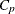## Overview: REG Procedure

The REG procedure is one of many regression procedures in the SAS System. It is a general-purpose procedure for regression, while other SAS regression procedures provide more specialized applications.

Other SAS/STAT procedures that perform at least one type of regression analysis are the CATMOD, GENMOD, GLM, LOGISTIC, MIXED, NLIN, ORTHOREG, PROBIT, RSREG, and TRANSREG procedures. SAS/ETS procedures are specialized for applications in time series or simultaneous systems. These other SAS/STAT regression procedures are summarized in Chapter 4: Introduction to Regression Procedures, which also contains an overview of regression techniques and defines many of the statistics computed by PROC REG and other regression procedures.

PROC REG provides the following capabilities:

• multiple MODEL statements

• nine model-selection methods

• interactive changes both in the model and the data used to fit the model

• linear equality restrictions on parameters

• tests of linear hypotheses and multivariate hypotheses

• collinearity diagnostics

• predicted values, residuals, studentized residuals, confidence limits, and influence statistics

• correlation or crossproduct input

• requested statistics available for output through output data sets

Nine model-selection methods are available in PROC REG. In the simplest method, PROC REG fits the complete model that you specify. The other eight methods involve various ways of including or excluding variables from the model. You specify these methods with the SELECTION= option in the MODEL statement.

The methods are identified in the following list and are explained in detail in the section Model-Selection Methods.

NONE

no model selection. This is the default. The complete model specified in the MODEL statement is fit to the data.

FORWARD

forward selection. This method starts with no variables in the model and adds variables.

BACKWARD

backward elimination. This method starts with all variables in the model and deletes variables.

STEPWISE

stepwise regression. This is similar to the FORWARD method except that variables already in the model do not necessarily stay there.

MAXR

forward selection to fit the best one-variable model, the best two-variable model, and so on. Variables are switched so that R square is maximized.

MINR

similar to the MAXR method, except that variables are switched so that the increase in R square from adding a variable to the model is minimized.

RSQUARE

finds a specified number of models with the highest R square in a range of model sizes.

finds a specified number of models with the lowestin a range of model sizes.